## Main

The CMS and ATLAS experiments at the Large Hadron Collider (LHC) have reported the discovery of a new boson1,2,3 with a mass near 125 GeV and with production rates, decay rates and spin-parity quantum numbers10,11,12,13,14 compatible with those expected for the standard model Higgs boson4,5,6,7,8,9. In the standard model, the Higgs boson is a spin-zero particle predicted to arise from the Higgs field which is responsible for electroweak symmetry breaking17,18. As such, the standard model Higgs boson couples directly to the W and Z bosons, and indirectly to photons. To date, significant signals have been reported in channels where the boson decays to either γγ, WW, or ZZ boson pairs11,12,13, as predicted by the theory. Overall, these results directly demonstrate that the new particle is intimately related to the mechanism of spontaneous electroweak symmetry breaking, whereby the W and Z bosons become massive, and thus it has been identified as a Higgs boson.

The standard model also predicts that the Higgs field couples to fermions through a Yukawa interaction, giving rise to the masses of quarks and leptons. The structure of the Yukawa interaction is such that the coupling strength between the standard model Higgs boson and a fermion is proportional to the mass of that fermion. As the masses of many quarks and leptons are sufficiently well known from experiment, it is possible within the standard model to accurately predict the Higgs boson decay rates to these fermions. The existence of such decays and the corresponding rates remain to be established and measured by experiment. Indirect evidence for the Higgs coupling to the top quark, an up-type quark and the heaviest elementary particle known to date, is implied by an overall agreement of the gluon–gluon fusion production channel cross-section with the standard model prediction. However, the masses of down-type fermions may come about through different mechanisms in theories beyond the standard model19. Therefore, it is imperative to observe the direct decay of this new particle to down-type fermions to firmly establish its nature. As a consequence of the Yukawa interaction discussed above, the most abundant fermionic Higgs boson decays will be to third-generation quarks and leptons, namely the bottom quark and the τ lepton, as the decay of a Higgs boson with a mass around 125 GeV to top quarks is kinematically not allowed. Therefore, the most promising experimental avenue to explore the direct coupling of the standard model Higgs boson to fermions is in the study of the decay to bottom quark–antiquark pairs (denoted as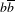) as well as to tau lepton–antilepton pairs (denoted as ττ).

Recently, the CMS Collaboration reported on a search for the decays of the new boson toquark pairs15 as well as to ττ lepton pairs16 based on data collected in 2011 and 2012. In this Letter, we report on the combination of the results from the study of these two decays to down-type fermion–antifermion pairs, performed for the first time at the LHC.

The CMS apparatus comprises several detectors specialized in identifying different types of particles. These detectors are arranged inside and outside a superconducting solenoid of 6 m internal diameter that provides a magnetic field of 3.8 T. The detector electronics process collision information at a rate of up to 40 MHz, and decide whether or not the crossing of proton beam bunches that took place at the centre of the detector produced proton–proton collisions of sufficient interest. Through a layered decision system, from the 20 MHz of proton bunch crossings, fewer than 1 kHz are saved for further analysis. A detailed description of the full CMS apparatus can be found in ref. 20. Data were collected at the LHC which delivered proton–proton collisions at centre-of-mass energies of 7 TeV (2011) and 8 TeV (2012). A total integrated luminosity of 5.1 (4.9) fb−1 and 18.9 (19.7) fb−1, for the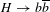(Hττ) decay, has been analysed at 7 and 8 TeV, respectively. Each proton bunch crossing gave rise to a large number of simultaneous proton–proton collisions, on average 9 in 2011 and 21 in 2012. Such a large number of overlapping collisions presented exceptional challenges in reconstructing the individual particles produced in the collision where an interesting interaction took place. Those challenges were successfully met thanks to the CMS tracking system, able to separate collision vertices as close as 0.5 mm along the beam direction.

The Higgs boson lifetime is 10−22 s. As a consequence, the detectors at the LHC only record the interactions of its decay products. For a Higgs boson mass of 125 GeV, the channels expected to be experimentally accessible include the decays to two photons, two W or Z bosons, aquark pair and a ττ lepton pair. The last two are especially challenging as the backgrounds due to standard model processes other than Higgs boson production are overwhelming and the invariant mass distributions of the reconstructed decay products do not produce sharp peaks. Techniques to estimate the background contributions directly from the collision data have been developed to face this challenge. The results are typically expressed in terms of upper limits on the product of the production and decay rates, significances for the background-only hypothesis to describe excesses as large, or larger, than those present in the data, and signal strengths relative to the expectation for the production and decay of the standard model Higgs boson. Both the observations in data and the expectations for the standard model Higgs boson are quoted. The expected quantities are important measures of the sensitivity of a particular search which do not depend on statistical fluctuations affecting actual observations in the data. Thus, they can also be used to compare the sensitivity of different experiments.

The CMS search for a Higgs boson decaying into aquark pair is reported in ref. 15. The inclusive production of Higgs bosons in this decay channel is extremely hard to exploit owing to the overwhelming direct production ofquark pairs, as predicted by quantum chromodynamics (QCD). For this reason, present searches target the so-called ‘VH production’ mode, in which the Higgs boson is produced in association with a heavy vector boson, V, namely a W or a Z boson, identified via its leptonic decay. Requiring the presence of additional leptons with high transverse momentum provides an effective handle to reduce the QCD background. The Higgs boson candidate is reconstructed from two jets of particles, each of which has been identified as having a high probability of arising from the hadronization of a bottom quark. The identification is based on properties of hadrons containing bottom quarks, such as their long lifetimes, which lead to displaced vertices, or their decay to muons, both producing distinctive signatures that the CMS detector is sensitive to. Owing to the 10% mass resolution of the identified pair of b-quark jets15, the standard model Higgs boson signal is expected to produce a broad enhancement over the background in theinvariant mass distribution. The result of this analysis shows an excess over the background expectation for Higgs boson mass hypotheses, mH, in the range of 120–135 GeV, with an observed (expected for the standard model Higgs boson) significance of 2.1 (2.1) standard deviations, σ, at a Higgs boson mass of 125 GeV. Correspondingly, the strength of this excess relative to the expectation for the standard model Higgs boson is 1.0 ± 0.5, where the uncertainty includes the effect of both statistical and systematic sources of uncertainty. A result obtained by the CDF and D0 experiments at the Tevatron proton–antiproton collider, which operated at a centre-of-mass collision energy of 1.96 TeV, initially revealed an excess ofevents consistent with the mass of the Higgs boson observed at the LHC, yielding an observed significance of 2.8σ for mH = 125 GeV (ref. 21). In a subsequent analysis the observed significance decreased, with the CDF and D0 experiments reporting an excess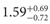times the expectation for the standard model Higgs boson with mH = 125 GeV (ref. 22). At the LHC, in previous studies based solely on the 2011 data, the CMS and ATLAS Collaborations have reported 95% confidence level (CL) upper limits of 2.5–5.5 times the standard model expectation for a Higgs boson in the 110–130 GeV mass range23,24.

Evidence for a Higgs boson decaying to a ττ lepton pair is reported by the CMS Collaboration in ref. 16. The lifetime of the τ lepton is 2.9 × 10−13 s, short enough that τ leptons decay before reaching any of the CMS detector systems. The predominant decay modes of τ leptons are to a lighter lepton (that is, an electron or a muon) and two neutrinos, or to one or more pions and a neutrino25. In all cases, among the decay products there are neutrinos which cannot be directly detected. However, the presence of neutrinos among the products of an interaction can be inferred from an overall imbalance in the transverse momentum, measured using the visible products of the interaction. The τ-lepton decays involving pions are dominated by the decays to a single charged pion, a charged and a neutral pion, or three charged pions observed as a collimated jet of particles. All of these decay modes can be identified by the CMS detector, providing an important handle to suppress the QCD background. The main irreducible background is due to the copiously produced Z bosons which, like the Higgs boson, can decay to a ττ lepton pair. The mass difference between the Z boson (91.2 GeV) and the region where a Higgs boson was observed (125 GeV), together with a dedicated ττ mass reconstruction technique16, allow a good separation of the two contributions. The gluon–gluon fusion production, the associated VH production, and the vector–boson fusion production processes are probed through the classification of the events according to kinematic and topological properties of the reconstructed particles. The result of the analysis shows a broad excess over background in the Higgs boson mass range of 115–130 GeV with an observed (expected) significance of 3.2σ (3.7σ) at a Higgs boson mass of 125 GeV. Correspondingly, the signal strength relative to the expectation for the standard model Higgs boson is found to be 0.78 ± 0.27 at the Higgs boson mass of 125 GeV. Earlier, the CDF and D0 experiments at the Tevatron reported, for mH = 125 GeV, an observed (expected) 95% CL upper limit of 7.0 (5.7) times the standard model expectation22. Using the LHC data taken at 7 TeV, the ATLAS and CMS Collaborations reported, for mH = 125 GeV, observed (expected) 95% CL upper limits of 3.7 (3.5; ref. 26) and 4.2 (3.1; ref. 27) times the standard model expectation, respectively.

The CMS measurements in theand Hττ searches15,16 are both consistent, within the precision of the present data, with each other and with the expectation for the production and decay of the standard model Higgs boson. Hence, as a next step, a combination of these two results, requiring the simultaneous analysis of the data selected by the two individual measurements, is performed. Such a simultaneous fit to both datasets accounts for all statistical and systematic uncertainties and their correlations. The statistical methods used for the combination were developed by the ATLAS and CMS Collaborations in the context of the LHC Higgs Combination Group. The presence of the signal is assessed by performing a fit to the observed data and using a test statistic based on the profile likelihood ratio. Systematic uncertainties are incorporated through nuisance parameters and are treated according to the frequentist paradigm. A detailed description of the methodology can be found in refs 28, 29 and results presented here are obtained using asymptotic formulae30. To quantify an excess of events over the background expectation, the test statistic is defined as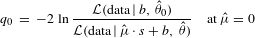where the likelihood,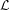, appearing in the numerator is that of the background-only hypothesis, s stands for the signal expected under the standard model Higgs boson hypothesis, μ is a signal strength modifier introduced to accommodate deviations from standard model Higgs boson predictions, b stands for background contributions, and θ are nuisance parameters describing the systematic uncertainties. The value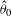maximizes the likelihood in the numerator under the background-only (μ = 0) hypothesis, while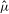anddefine the point at which the likelihood reaches its global maximum in the signal-plus-background fit. The post-fit model, obtained after the signal-plus-background fit to the data, is used when deriving expected quantities. The post-fit model corresponds to the parametric bootstrap described in the statistics literature, and includes information gained in the fit regarding the values of all parameters31,32. The p-value for the background-only hypothesis is calculated as the tail probability p0 = P(q0q0obs.|b), where q0obs. is the value of the test statistic observed in data.

Whereas the expected Higgs boson signal in the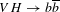analysis consists exclusively of Higgs bosons decaying to down-type fermions, that is not the case in some event categories in the Hττ analysis where a sizeable contribution from HWW decays is expected. The contribution from HWW decays has kinematic properties sufficiently different from those of Hττ decays such that the two contributions do not overlap. To exclusively assess the fermionic decays of a Higgs boson, the expected contribution from HWW decays is considered as a background process. Given the discovery of a Higgs boson with properties as expected in the standard model and a mass close to 125 GeV, the ppHWW background contribution is taken from the expectation for the standard model Higgs boson with a mass of 125 GeV, including all associated uncertainties.

Figure 1 shows, as a function of the mass hypothesis for the Higgs boson decaying to fermions, the median expected and the observed p-value for the background-only hypothesis. The expectation is calculated after having performed the signal-plus-background fit to the observed data. The background-only hypothesis includes the ppH(125 GeV) →WW process for every value of mH. The p-value can be expressed in terms of Gaussian tail probabilities and given in units of standard deviation (σ ), shown in the right vertical axis of Fig. 1. For all mH values tested, the evidence against the background-only hypothesis is found to be 3σ or more, with a maximum of 3.8σ for mH = 125 GeV, corresponding to a p-value of 7.3 × 10−5.Figure 1: The p-value for the background-only hypothesis as a function of the Higgs boson mass hypothesis for both the observed data (obs.) and pseudo-data constructed using the median expectation for the standard model Higgs boson after the signal-plus-background fit to the data (exp.).

The study of the fermionic-decay contribution under the mH = 125 GeV hypothesis is particularly relevant because of the discovery of a Higgs boson with a mass near 125 GeV in the analysis of non-fermionic decays. As the mass resolution in theand Hττ channels is, at best, around 10%, and the expected yields do not change significantly around mH = 125 GeV, the exact choice of mH does not affect the conclusions. A scan of the profile likelihood is performed to estimate the best-fit signal strength relative to the standard model expectation, as well as confidence intervals. As described earlier, the HWW contribution is not treated as part of the signal. The result is shown in Fig. 2, from which a combined signal strength of 0.83 ± 0.24 with respect to the standard model expectation is inferred for the production of a Higgs boson decaying to down-type fermions. The statistical component of the uncertainty represents 80% of the total uncertainty. This result is compatible with the expectation for the standard model Higgs boson, as summarized in Table 1.Figure 2: Scan of the profile likelihood as a function of the signal strength relative to the expectation for the production and decay of a standard model Higgs boson, μ, for mH = 125 GeV.

In conclusion, the existing CMS searches for a Higgs boson decaying into bottom quarks and τ leptons are consistent with the standard model prediction of a Yukawa structure, where the fermionic couplings are proportional to the fermion masses. Combining the results of the CMS measurements in theand Hττ decay channels and assuming that the Higgs boson is produced as expected in the standard model, reveals, for the first time, strong evidence for the direct coupling of the 125 GeV Higgs boson to down-type fermions, with an observed (expected) significance of 3.8 (4.4) standard deviations.